# Eisenstein cohomology

The basic ingredients for Eisenstein cohomology are a reductive group, an arithmetic subgroup (cf. also Arithmetic group)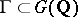and a rational representation(cf. also Representation of a group). The simplest example is given by the group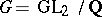, a congruence subgroup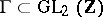with as representation the space of homogeneous polynomials of degree,where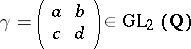acts by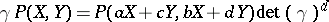.

Starting from these data one can construct a symmetric space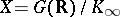, the locally symmetric space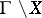and a sheaf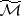on. In the example one can take, whereis the connected component of the identity of the real points of the centre.

One can consider the cohomology groups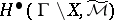. It is a consequence of reduction theory that they form a finite-dimensional (graded) vector space. It carries some further structure: It can be endowed with an action of the so-called Hecke algebra on it. It is a fundamental problem to understand these cohomology groups as a module under this action of the Hecke algebra.

Ifis torsion free, thenis a Riemannian manifold with finite volume and sometimes it even carries the structure of a quasi-projective variety. If, for instance,, thenis a compact Riemann surface with a finite non-empty set of points removed.

Form the tensor product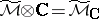. A method for investigating these cohomology groups is provided by the de Rham isomorphismwhere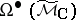is the de Rham complex of, and this de Rham complex is isomorphic towhere(respectively,) is the Lie algebra of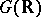(respectively,).

This opens the door for the application of representation-theoretical methods, becauseis a module under the group.

However, since the quotientis not compact in general, one has to be careful: the space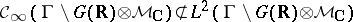and the Hilbert space technique of decomposing a module into irreducibles cannot be applied so easily. It is possible to define various subspaces, namely the space of cusp forms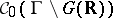, or the space of compactly supported functions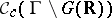. These define subspaces in the cohomology, namely the cuspidal cohomology and the so-called "inner cohomology"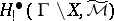. Classes in one of theses subspaces vanish "at infinity" . The cuspidal cohomology can be investigated using classical Hilbert space techniques; the spaceis a countable sum of irreducible subspaces under the action of.

One wants to understand the rest of the cohomology. To that end one can embed the locally symmetric spaceinto the Borel–Serre compactification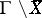; the sheaf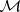extends to a local system on this compactification. The Borel–Serre compactification is a manifold with corners; let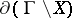be its boundary. Then there is an exact sequence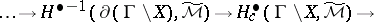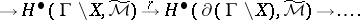This raises the question to understand such sequences: What is the cohomology of the boundary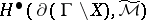and what is the image of the "global" cohomologyin the cohomology of the boundary?

Understanding the cohomology of the boundary requires understanding the cohomology of groups of lower rank, which is sometimes not so difficult. For instance, in the special example above the boundary consists of a finite numberof circles and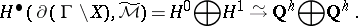Eisenstein cohomology is designed to provide some understanding of the restriction mapping. One starts from a class(or a certain space of classes) in the cohomology of the boundary. Viewing it as a class in the de Rham cohomology, one can represent it by a form which is not invariant under, but which is invariant under the smaller group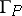given by the intersection ofwith a parabolic subgroupwhich enters in the datum. Then one forms the sumgiving a global class. The only difficulty is that this sum need not converge. Hence the formhas to be "twisted" by a parametervarying in a space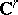and whose real part should be large. Then the sum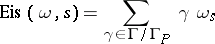will be convergent and represent a holomorphic function in. Langlands' general theory of Eisenstein series implies that this function has a meromorphic continuation and hence one can "evaluate" at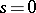. However, various things may happen. One may encounter a pole or the class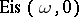need not be closed. If it is closed, one has to compute its restriction to the boundary.

What happens exactly depends, of course, on the original data. The original formshould be specified more precisely; for instance, one may assume that it is an eigenform for a certain subalgebra of the Hecke algebra. Then as such it produces certain-functions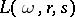(cf. also-function) and the answer to the above questions depends on the behaviour of certain of these-functions at.

This program has been carried out successfully in some low-dimensional cases, see, for instance, [a2], [a3], [a7]. In the example, the restriction mappingis surjective in degree one and zero in degree zero if. If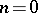, then the image ofin degree one has codimensionand dimensionin degree zero. Actually, in this case the theory of Eisenstein series is not needed, since purely topological arguments are sufficient.

It has been demonstrated in [a2], [a3], [a7] that a detailed understanding of the Eisenstein cohomology may have certain arithmetic implications; for instance, one obtains rationality results for special values of-functions. One may also hope that via the influence of the values of the-functions on the structure of the cohomology as a module under the Hecke algebra, some interesting arithmetic objects (mixed motives, unramified field extensions) can be constructed that owe their existence to the (arithmetic) properties of certain-values; see [a4], [a5], [a6].

Finally, there is the following fundamental and very general theorem of J. Franke [a1]. Using the Eisenstein series and their residues and derivatives one can define the subspace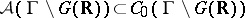. This space can also be characterized as a space of functions that satisfy certain growth conditions and differential equations.

This subspace is "very small" and Franke's theorem says that the mappinginduces an isomorphism in cohomology.